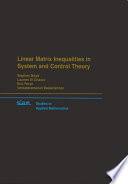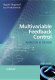### Applied Optimal Control

#### Course Description

Optimal control of linear and linear parameter varying systems. Application of convex optimization and linear matrix inequalities in optimal controller synthesis. Application of a reference governor for handling constraints in existing control systems.

#### Learning Outcomes

1. Formulate linear parameter varying model of the system
2. Apply linear matrix inequalities for optimal controller synthesis
3. Design a referece governor to handle contstraints on an existing control system

#### Forms of Teaching

Lectures

Lectures will be given on weekly basis with weekly load of 2 hours

Laboratory

Wirhin the course 4 three-hours laboratory exams will be organized

#### Week by Week Schedule

1. Optimal controller design based on integral criteria, Parametric optimization in control systems
2. Convex optimization in control systems
3. Linear matrix inequalities - basic definitions and properties
4. Stability condition and decay ratio maximization
5. Constraints on control inputs and outputs
6. Pole placement controller design using LMI
7. H2/Hinf controller design using LMI
8. Midterm exam
9. Linear parameter varying (LPV) systems - basic definitions and properties, Modelling of LPV systems
10. Common Lyapunov function based control of LPV systems
11. Parameter varying Laypunog function based control of LPV systems
12. Reference governor (RG) for linear systems (scalar RG, vector RG)
13. Reference governor for nonlinear systems (linearization based, Lyapunov function based)
14. Robust reference governor
15. Final exam

#### Literature(.), Boyd, S., El Ghaoui, L., Feron, E., &amp;amp;amp;amp;amp; Balakrishnan, V. (1994). Linear matrix inequalities in system and control theory (Vol. 15). Siam.,
(.), Scherer, C., &amp;amp;amp;amp;amp; Weiland, S. (2000). Linear matrix inequalities in control. Lecture Notes, Dutch Institute for Systems and Control, Delft, The Netherlands, 3(2).,
(.), Boyd, S., & Vandenberghe, L. (2004). Convex optimization. Cambridge university press.,(.), Skogestad, S., & Postlethwaite, I. (2007). Multivariable feedback control: analysis and design (Vol. 2, pp. 359-368). New York: Wiley.,

#### General

ID 222703
Winter semester
5 ECTS
L3 English Level
L1 e-Learning
30 Lectures
12 Laboratory exercises